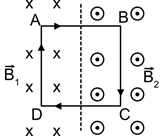A square frame, made of copper wire is carrying a current i=12 A. Length of side of the square is 1 m. If the frame is placed in a magnetic region where B1=1 μT and B2=2 μT, then net force experienced by the square frame is

# A square frame, made of copper wire is carrying a current . Length of side of the square is 1 m. If the frame is placed in a magnetic region where  then net force experienced by the square frame is1. A

2. B

3. C

4. D

Register to Get Free Mock Test and Study Material

+91

Verify OTP Code (required)